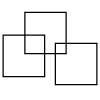#### You may also like### Three Squares

What is the greatest number of squares you can make by overlapping three squares?### Two Dice

Find all the numbers that can be made by adding the dots on two dice.### Biscuit Decorations

Andrew decorated 20 biscuits to take to a party. He lined them up and put icing on every second biscuit and different decorations on other biscuits. How many biscuits weren't decorated?

# Fair Feast

##### Age 5 to 11Challenge Level

We have been inundated with solutions to this problem - thank you so much! It is very difficult to pick some names to mention. Deiniol explained:

I found the answer by dividing the total number of each item of food by 2 (because there were two boys!). Each boy will have - 2 slices of pizza, 4 tomatoes, a carton of orange juice, half an apple and 2 muffins.

Year 2 Oak Class at Twyford St Mary's have been learning about fractions and the teacher wrote,

We have had a go at the Fair Feast problem and here is our solution for you.
We used what we know about fractions, halving numbers and division to find out what would happen if 4 people shared this picnic.
If we share between four people each person would get 1 slice of pizza or 1/4 of the pizza. They would get 1 muffin each because there are 4 and that shares easily by 4 people. They could also have two halves of a muffin, because that is the same as one whole. For the tomatoes, we used division and said that 8 divided by 4 equals 2, so they each get 2. The apple would be equally cut into 4 slices or quarters. Each person would have 1 quarter as that is 1 out of 4. If the carton was 200ml, each person would get 100ml because half of 200 is 100. Two people would share one carton.
Now we challenge other children to try and find out if the picnic can be shared by 8, 10, 12 or 20 people!

Anna and Eden from West Hill Primary wrote:

They would split the apple in half. They would have half a pizza each, two muffins each, four tomatoes each and one drink each.

Of course this is exactly the same as Deiniol's solution, but the amount of pizza is given in a slightly different way.

Alisha and Madalena at Victoria Road Primary sent a very clear solution:

We found out that we had to split all the food and drink in to half because there are two people.

We know they get half a pizza each and this is 2 slices because the pizza is cut in quarters and we know that two quarters is the same as a half.

They would get 4 tomatoes each because half of 8 is 4.

Half of an apple each because they only have 1 apple and 1 whole cut in to 2 is half.

2 muffins each because half of 4 is 2.

1 juice each because half of 2 is 1.

Children from Oakfield Prep School drew pictures to help them work out how much picnic each boy would get.  You can see their pictures in the following files:

Henry.pdf
Harris_Zain.pdf
Ryan.pdf